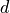# Particle¶

class `halmd.mdsim.``particle`(args)

Construct particle instance.

Parameters: args (table) – keyword arguments args.dimension (number) – dimension of space args.particles (number) – number of particles args.species (number) – number of species (default: 1) args.memory (string) – device where the particle information is stored (optional) args.precision (string) – floating point precision (optional) args.label (string) – instance label (default: `all`)

The supported values for `memory` are `host` and `gpu`. If `memory` is not specified, the memory location is selected according to the compute device.

The supported values for `precision` are `single` and `double-single` if `memory` equals `gpu`, and `double` for host memory. If `precision` is not specified, the highest available precision is used.

`nparticle`

Number of particles.

`nspecies`

Number of particle species.

`memory`

Device where the particle memory resides.

`precision`

Floating-point precision of the data stored.

`label`

Instance label.

`data`

```table = particle.data["position"]
particle.data["position"] = table
```

or using the equivalent syntax:

```table = particle.data.position
particle.data.position = table
```

The following named arrays holding per-particle data are predefined:

• scalar integer data:
• `id`: particle ID, unique within instance of `halmd.mdsim.particle`
• `reverse_id`: maps a particle ID to its current array index in memory
• scalar floating-point data:
• vector integer data:
• `image`: periodic image of the simulation box that contains the given particle
• vector floating-point data:
• `force`: force acting on each particle
• `position`: position reduced to the original periodic simulation box
• `velocity`: velocity of each particle
• other floating-point fields:
• `potential_stress_tensor`: contribution to the potential part of the stress tensor. Indimensions,diagonal entries of this symmetric tensor are followed byoff-diagonal entries.

Warning

Accessing particle data using this table is equivalent to calling `get()` and `set()`, respectively, and involves a full copy of the data to a lua table.

Warning

During simulation, particle arrays may be reordered in memory according to a space-filling curve, see `halmd.mdsim.sorts`. To access particles in initial order, use `get_reverse_id()` to retrieve a map from particle IDs to current particle indices in memory.

`get`(name)

Returns particle data identified by the name of the particle array.

Parameters: name (string) – identifier of the particle array
`set`(name, data)

Set particle data identified by the name of the particle array.

Parameters: name (string) – identifier of the particle array data (table) – table containing the data
`shift_velocity`(vector)

Shift all velocities by `vector`.

`shift_velocity_group`(group, vector)

Shift velocities of group by `vector`.

`rescale_velocity`(scalar)

Rescale magnitude of all velocities by `scalar`.

`rescale_velocity_group`(group, scalar)

Rescale magnitude of velocities of group by `scalar`.

`shift_rescale_velocity`(vector, scalar)

First shift, then rescale all velocities.

`shift_rescale_velocity_group`(group, vector, scalar)

First shift, then rescale velocities of group.

`aux_enable`()

Enable the computation of auxliliary variables in the next on_force() step. These are: `stress_pot` and `potential_energy` and derived properties (such as the internal energy or the virial). The auxiliary variables should be activated like this:

```sampler:on_prepare(function() particle:aux_enable() end, every, start)
```
`on_prepend_force`(slot)

Connect nullary slot to signal.

Returns: signal connection
`on_force`(slot)

Connect nullary slot to signal.

Returns: signal connection
`on_append_force`(slot)

Connect nullary slot to signal.

Returns: signal connection
`__eq`(other)
Parameters: other – instance of `halmd.mdsim.particle`

Implements the equality operator `a = b` and returns true if the other `particle` instance is the same as this one.